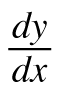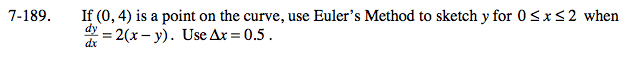### Home > CALC > Chapter 7 > Lesson 7.4.2 > Problem7-189

7-189.

If (0, 4) is a point on the curve, use Euler's Method to sketch y for 0 ≤ x ≤ 2 when= 2(xy). Use Δx = 0.5. Homework Help ✎Notice that you are given the derivative equation. That means you can calculate the slope at any given point.

So if you were to draw a line segment starting at (0, 4) with slope of −8, what would be the coordinate point at x = 0.5?

Use the coordinate point (0.5, ______) to calculate a new slope and find the coordinate point at x = 1.
Do the same to find the coordinate at x = 1.5
And again at x = 2.

(2, 1.5)